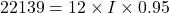Question

Assuming 95.0% efficiency for the conversion of electrical power by the motor, what current must the 12.0-V batteries of a 708-kg electric car be able to supply to do the following?

(a) accelerate from rest to 25.0 m/s in 1.00 min

(b) climb a 200-m high hill in 2.00 min at a constant 25.0 m/s speed while exerting 423 N of force to overcome air resistance and friction

1.(a). The current is 323.4 A.

(b). The current is 1942 A.

Explanation:

Given that,

Efficiency = 95.0 %

Voltage = 12.0 V

Mass of electric car= 708 Kg

Height = 200 m

We need to calculate the change in kinetic energy

Using formula of kinetic energy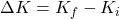Put the value into the formula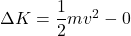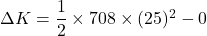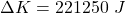We need to calculate the output power

Using formula of power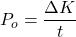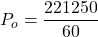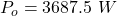We need to calculate the current

Using formula of electric power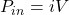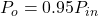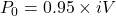Put the value into the formula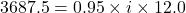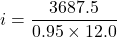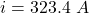The current is 323.4 A.

(b). We need to calculate the distance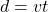Put the value into the formula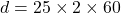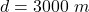We need to calculate the force

Using formula of force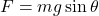Put the value into the formula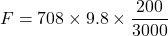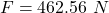We need to calculate the power

Using formula of power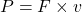Put the value into the formula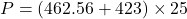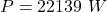We need to calculate the current

Using formula of current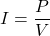Put the value into the formula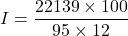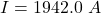The current is 1942 A.

Hence, (a). The current is 323.4 A.

(b). The current is 1942 A.

2.quangkhai

a)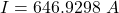b)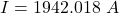Explanation:

Given:

• efficiency of the motor,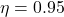• voltage of the battery,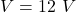• mass of the car,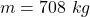a)

initial velocity,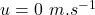final velocity,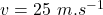time taken for the acceleration,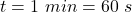Now we know by the Newton’s second law of motion: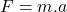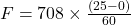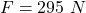Now the power will be :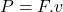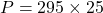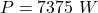According to the question:

0.95 times of the electrical power should yield this mechanical power.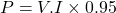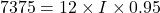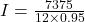b)

height climbed by the car,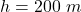velocity of climb,time taken to climb the height,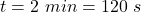force exerted to overcome air and frictional resistances,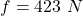Now the Power required to climb the hill: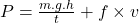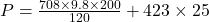Now according to the electrical efficiency: